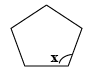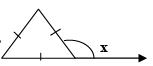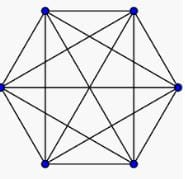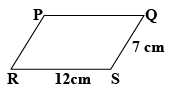# Test: Practical Geometry- 2

## 40 Questions MCQ Test NCERT Mathematics for CAT Preparation | Test: Practical Geometry- 2

Description
Attempt Test: Practical Geometry- 2 | 40 questions in 40 minutes | Mock test for Class 8 preparation | Free important questions MCQ to study NCERT Mathematics for CAT Preparation for Class 8 Exam | Download free PDF with solutions
QUESTION: 1

### A simple closed curve made up of only _____________ is called a polygon .

Solution:
A simple closed curve made of three or more line-segments is called a polygon. A polygon has at least three line-segments.
The line-segments of a polygon are named by the sides in it. Poly means many and gon means sides. Thus a polygon has many sides.
QUESTION: 2

Solution:
QUESTION: 3

### Polygons that have no portions of their diagonals in their exteriors are called

Solution:

Convex polygons have each interior angle less than 180, so its vertices point outside. So there are no exterior diagonals in convex polygons.

QUESTION: 4

Polygons that have any portions of their diagonals in their exteriors are called

Solution:
QUESTION: 5

All the sides of a regular polygon are _________________.

Solution:
QUESTION: 6

All the angles of a regular polygon are of ________________.

Solution:
QUESTION: 7

Sum of all interior angles of a polygon with (n) sides is given by

Solution: Correct answer is option a because if we take the polygon triangle. the no.of side =3 so,the sum of its interior angle =180degree by this formula(n-2)×180degree 3-2×180degree =180degree so proved.
QUESTION: 8

Maximum number of right angles in a right angled triangle are

Solution: Because the sum of a triangle is 180 degree and of right triangle is 90 degree and if there is two right triangle then their sum is 180 degree this is not possible to have two right angle in a right angle triangle.
QUESTION: 9

Sum of all interior angles of a parallelogram  is

Solution:
QUESTION: 10

The angle sum of all interior angles of a convex polygon of sides 7 is

Solution:
QUESTION: 11

Each exterior angle of a regular hexagon is of measure

Solution:
QUESTION: 12

The number of sides in a regular polygon is15, then measure of each exterior angle is

Solution: The formula of each exterior angle is 360/no.of sides so 15=360/n, then n = 360/15=24degree.
QUESTION: 13

The measure of each interior angle of a regular polygon is 140o, then number of sides that regular polygon has ___

Solution:

The corresponding exterior angle must be 180-140 = 40 degrees

The sum of ALL the exterior angles is ALWAYS 360.

exterior angles = 360/40 = 9

Therefore, number of sides = 9

QUESTION: 14

Which of the following polygons is convex polygon ?

Solution:
QUESTION: 15

Which of the following is concave polygon?

Solution:
QUESTION: 16

To construct a parallelogram we need to know:

Solution:

Hint: Parallelogram has its parallel sides equal. Also, if one angle is known to us, then we can determine the other angle since the two angles are supplementary.

QUESTION: 17

A quadrilateral which has 2 pairs of equal adjacent sides but unequal opposite sides is called ________________.

Solution:
QUESTION: 18

The value of x in the following figure is:Solution:

Each interior angle of a regular pentagon is 108o

QUESTION: 19

The value of x in the following figure isSolution:
QUESTION: 20

A parallelogram each of whose angles measures 90o is _____________.

Solution:
QUESTION: 21

A parallelogram whose all sides are equal is called

Solution:

Rhombus is a type of parallelogram whose all sides are equal

QUESTION: 22

The diagonals of a rhombus bisect each other at  _________  angles.

Solution:

The diagonals of a rhombus are perpendicular bisectors of each other.

QUESTION: 23

Diagonals of a rectangle:

Solution:

Take a rectangle with 2 diagonals and take 2 triangles who include different diagonals . the triangles can be proved congruent and hence the diagonals can be proved equal.

QUESTION: 24

The diagonals of a square bisect each other at  _________  angle.

Solution:
QUESTION: 25

The least number that is divisible by all the numbers from 1 to 10 (both inclusive) is

Solution:
QUESTION: 26

Minimum possible interior angle in a regular polygon is ___________.

Solution:
QUESTION: 27

Maximum possible exterior angle in a regular polygon is ___________.

Solution:
QUESTION: 28

How many sides does a heptagon have ?

Solution:
QUESTION: 29

Name the closed figure with 4 sides ?

Solution:
QUESTION: 30

How many diagonals does a regular Hexagon has?

Solution:QUESTION: 31

What is the number of sides in Hexagon ?

Solution:
QUESTION: 32

What is the sum of the measures of angles of a convex quadrilaterals?

Solution:
QUESTION: 33

If the three angles of a quadrilateral are 120o, 130o, 10o then what is the fourth angle ?

Solution:
QUESTION: 34

The opposite angles of a parallelogram are  __________________.

Solution:
QUESTION: 35

The perimeter of parallelogram PQRS is:Solution:

Perimeter of a parallelogram =sum of all sides. Since opposite sides of a parallelogram are equal, perimeter of parallelogram is 12+12+7+7=38cm

QUESTION: 36

The diagonals of a square are ________________ each other.

Solution: As the diagonals bisected each other i.e equal And the point of bisection is 90 degrees.
QUESTION: 37

If in a parallelogram its diagonals bisect each other and are equal then it is a,

Solution:
A rhombus is a type of parallelogram, and what distinguishes its shape is that all four of its sides are congruent.

QUESTION: 38

How many measurements can determine a quadrilateral uniquely?

Solution: In a parallelogram, opposite sides are equal, opposite angles are equal and diagonals bisect each other. In a rhombus diagonals intersect at right angles. In a rectangle diagonals are equal. Five measurements can determine a quadrilateral uniquely.
QUESTION: 39

Diagonals of a parallelogram _______________ each other.

Solution:
QUESTION: 40

How many sides does decagon has?

Solution:Use Code STAYHOME200 and get INR 200 additional OFF Use Coupon Code